# Impulse of Jets Notes | Study Mechanical Engineering SSC JE (Technical) - Mechanical Engineering

## Mechanical Engineering: Impulse of Jets Notes | Study Mechanical Engineering SSC JE (Technical) - Mechanical Engineering

The document Impulse of Jets Notes | Study Mechanical Engineering SSC JE (Technical) - Mechanical Engineering is a part of the Mechanical Engineering Course Mechanical Engineering SSC JE (Technical).
All you need of Mechanical Engineering at this link: Mechanical Engineering

IMPULSE OF JETS

• Jet Strikes normal to the flat stationary plate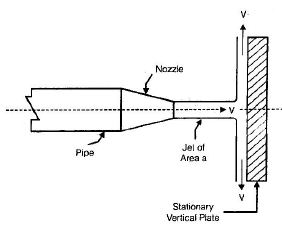Force exerted by the jet normal to the plate
P= ρaV2
a = area of jet
V = velocity of jet 

• Jet strikes on a inclined stationary plate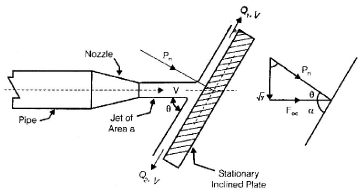• Force exerted by the jet normal to the plate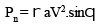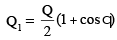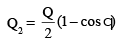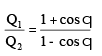•  Force exerted by jet on moving flat plate normal to jet.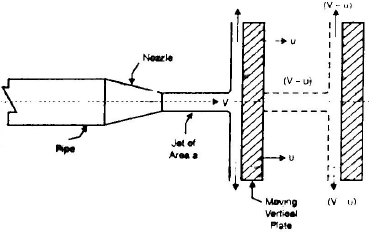u = plate velocity
Pn  = ρa(V – u)2
Work done per second (W) = Pn × u = ra[V – u]2 × u 

• Jet strikes on series of flat plat mounted on the periphery of wheel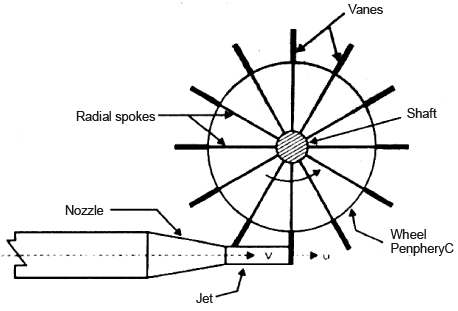P= ρaV(V – u)
Work done by the jet = Pn × U
W = ρaV(V – u)u

Efficiency of the work done of wheel h =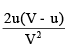at ,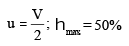•  Jet striking on a symmetrical stationary curved plate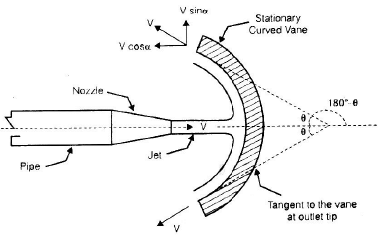Pn = ρaV2 (1 + cos q)
Force exerted by a jet in its direction of flow on a curved vane is always greater than that exerted on a flat plate.
Force Exerted By a jet on a Hinged Plate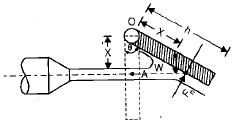•  Force due to jet of water, normal to the plate
Pn = ρaV2 sin (90º –θ) 
• For equilibrium of plate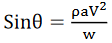The document Impulse of Jets Notes | Study Mechanical Engineering SSC JE (Technical) - Mechanical Engineering is a part of the Mechanical Engineering Course Mechanical Engineering SSC JE (Technical).
All you need of Mechanical Engineering at this link: Mechanical EngineeringUse Code STAYHOME200 and get INR 200 additional OFF

## Mechanical Engineering SSC JE (Technical)

6 videos|89 docs|55 tests

Track your progress, build streaks, highlight & save important lessons and more!

,

,

,

,

,

,

,

,

,

,

,

,

,

,

,

,

,

,

,

,

,

;